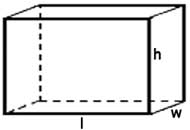# Rectangular Prism Volume Calculator

Measurements, inserted by the user, will be used by the online Rectangular Prism Volume Calculator to carry out the calculations of the volume electronically.

The user will also be provided with a running total which will accumulate as new dimensions are introduced into the Rectangular Prism Volume Calculator.## General information and basic definition of a rectangular prism

A rectangular prism is a prism with a rectangular base and faces that correspond to each side of the base.

A prism has the same cross section all along its length.

The following formula can be used to work out the Volume of a Rectangular Prism:

• Volume = Width × Length × Height

Note: It is important to apply similar measurement units for all of the dimensions during the process of calculation of the volume of an object.

The following units are used to measure the volumes of solid objects:

• feet cubed
• meters cubed
• yards cubed

The following units are used when calculating the volumes of liquids:

• liters
• quarts
• pints
• gallons